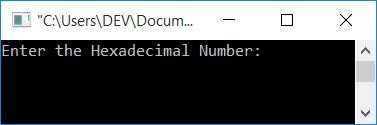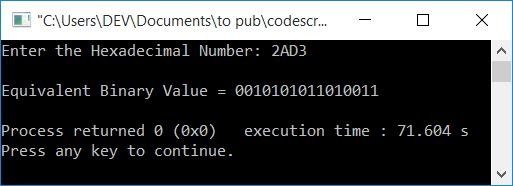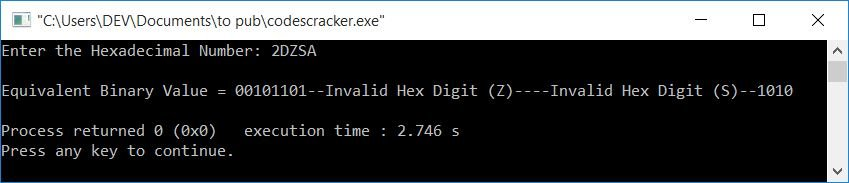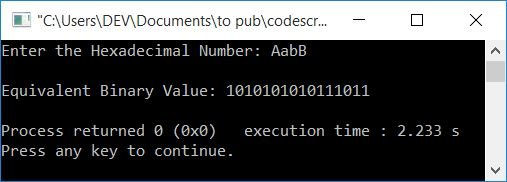# C++ Program to Convert Hexadecimal to Binary

In this article, you will learn and get code on hexadecimal to binary conversion in C++. But if you're not aware of the simple steps and formula used for the conversion, then you can refer to hexadecimal to binary to get all the required things.

## Hexadecimal to Binary in C++

To convert a hexadecimal number to a binary number in C++ programming, you have to ask the user to enter the hexadecimal number first. and then convert it into its equivalent binary value and print the equivalent binary value as output.

You can create the program in two ways:

• Either directly print the equivalent 4-bit binary value of each and every hexadecimal digit (hex digit) one by one, using switch case.
• Or use the strcat() function to concatenate each and every 4-bit binary equivalent of hex digit(s) in a variable, say binaryNum[], and then print the value of binaryNum[] at last. That is, first convert, then print.

### Print the binary equivalent of a given hexadecimal number in C++

This program uses switch case to match and print the binary equivalent of each and every hex digit.

```#include<iostream>
using namespace std;
int main()
{
int i=0;
char hexDecNum;
cin>>hexDecNum;
cout<<"\nEquivalent Binary Value = ";
while(hexDecNum[i])
{
switch(hexDecNum[i])
{
case '0':
cout<<"0000";
break;
case '1':
cout<<"0001";
break;
case '2':
cout<<"0010";
break;
case '3':
cout<<"0011";
break;
case '4':
cout<<"0100";
break;
case '5':
cout<<"0101";
break;
case '6':
cout<<"0110";
break;
case '7':
cout<<"0111";
break;
case '8':
cout<<"1000";
break;
case '9':
cout<<"1001";
break;
case 'A':
case 'a':
cout<<"1010";
break;
case 'B':
case 'b':
cout<<"1011";
break;
case 'C':
case 'c':
cout<<"1100";
break;
case 'D':
case 'd':
cout<<"1101";
break;
case 'E':
case 'e':
cout<<"1110";
break;
case 'F':
case 'f':
cout<<"1111";
break;
default:
cout<<"--Invalid Hex Digit ("<<hexDecNum[i]<<")--";
}
i++;
}
cout<<endl;
return 0;
}```

This program was built and runs under the Code::Blocks IDE. Here is its sample run:Now supply a hexadecimal number input, say 2AD3, and press the ENTER key to print its equivalent binary value as shown in the output given below:The following is a dry run of the above program with hexadecimal input, say 2AD3:

• Initial value, i=0
• When the user enters a hexadecimal number, say 2AD3, then it gets initialized to hexDecNum in a way that:
• hexDecNum=2
• hexDecNum=A
• hexDecNum=D
• hexDecNum=3
• Now the condition of the while loop gets evaluated. That is, the condition hexDecNum[i] or hexDecNum evaluates to be true because a value of 2 is available at hexDecNum.
• Because the condition evaluates to be true, the program flow goes inside the loop, and using switch, the character gets matched with all 15 cases.
• That is, it determines whether the character at hexDecNum[i] or hexDecNum is equal to one of the 15 character cases, such as 0, 1, 2, ...., A, a, B, ..., f, or not.
• If it equals any of the 15 characters, then print its equivalent 4-bit binary value. Otherwise, the default case gets executed.
• Now the value of i gets incremented, and the program flow goes back and evaluates the condition of the while loop again.
• When the value of i becomes equal to 4, then the condition hexDecNum evaluates to be false, because at the 4th index, no further character is available, rather, a null-terminated character (\0) is available at the 4th index.
• On continuing the process, we'll get the binary equivalent of the 2AD3 hexadecimal input as 0010101011010011. Where 2=0010, A=1010, D=1101, and 3=0011

#### What if the user enters an invalid hexadecimal digit?

In this case, if the user enters an invalid hexadecimal digit, For example, if the user enters hexadecimal input in the following ways (containing one or more invalid hex digits, with or without valid ones):

• Z: One invalid hexadecimal digit
• 2DZSA: An invalid hexadecimal digit, in between valid ones.
• TR: Two invalid hexadecimal digits

If the program (created above) finds any hexadecimal digit (regardless of where or how it appears), a message --Invalid Hex Digit (HexDigit)-- is printed in such a way that:

• For the first case
--Invalid Hex Digit (Z)--
gets printed
• For the second case
00101101--Invalid Hex Digit (Z)----Invalid Hex Digit (S)--1010
gets printed. Where 0010 and 1101 are the hexadecimal values of 2 and D, Finally, A's hexadecimal value is 1010.
• For the third case
--Invalid Hex Digit (T)----Invalid Hex Digit (S)--
gets printed

Here is the sample output for the second case:Note: The above program doesn't convert a given hexadecimal number into its equivalent binary value. It simply prints the binary equivalent using switch case.

Now let's create another program that actually converts the number from hexadecimal to binary.

### Convert Hexadecimal to Binary in C++

The strcat() function is used in this program to concatenate the binary equivalent of each and every hexadecimal digit (hex digit) one by one to a variable called binaryNum[]. And finally, it prints its value as output.

```#include<iostream>
#include<string.h>
using namespace std;
int main()
{
int i=0, chk=0;
char hexDecNum, binaryNum="";
cin>>hexDecNum;
while(hexDecNum[i])
{
switch(hexDecNum[i])
{
case '0':
strcat(binaryNum, "0000");
break;
case '1':
strcat(binaryNum, "0001");
break;
case '2':
strcat(binaryNum, "0010");
break;
case '3':
strcat(binaryNum, "0011");
break;
case '4':
strcat(binaryNum, "0100");
break;
case '5':
strcat(binaryNum, "0101");
break;
case '6':
strcat(binaryNum, "0110");
break;
case '7':
strcat(binaryNum, "0111");
break;
case '8':
strcat(binaryNum, "1000");
break;
case '9':
strcat(binaryNum, "1001");
break;
case 'A':
case 'a':
strcat(binaryNum, "1010");
break;
case 'B':
case 'b':
strcat(binaryNum, "1011");
break;
case 'C':
case 'c':
strcat(binaryNum, "1100");
break;
case 'D':
case 'd':
strcat(binaryNum, "1101");
break;
case 'E':
case 'e':
strcat(binaryNum, "1110");
break;
case 'F':
case 'f':
strcat(binaryNum, "1111");
break;
default:
chk = 1;
break;
}
i++;
}
if(chk==0)
cout<<"\nEquivalent Binary Value: "<<binaryNum;
else
cout<<endl;
return 0;
}```

Here is its sample run with a hexadecimal number input as AabB:#### The same program in different languages

C++ Quiz

« Previous Program Next Program »